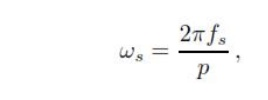Home | | Solid State Drives | V/F of Permanent Magnets Synchronous Motors

# V/F of Permanent Magnets Synchronous Motors

Constant volt per hertz control in an open loop is used more often in the squirrel cage IM applications. Using this technique for synchronous motors with permanent magnets offers a big advantage of sensor less control.

V/F OF PERMANENT MAGNETS SYNCHRONOUS MOTORSConstant volt per hertz control in an open loop is used more often in the squirrel cage IM applications. Using this technique for synchronous motors with permanent magnets offers a big advantage of sensor less control. Information about the angular speed can be estimated indirectly from the frequency of the supply voltage. The angular speed calculated from the supply voltage frequency ac- cording to (1) can be considered as the value of the rotor angular speed if the external load torque is nothing her than the break down torque.

The mechanical synchronous angular speed ωs is proportional to the frequency fs of the supply voltageWhere p is the number of pole pairs.

The RMS value of the induced voltage of AC motors is given asBy neglecting the stator resistive voltage drop and as sum- in steady state conditions, the stator voltage is identical to the induced one and the expression of magnetic flux can be written asTo maintain the stator flux constant at its nominal value in the base speed range, the voltage-to-frequency ratio is kept constant, hence the name V/f control. If the ratio is different from the nominal one, the motor will become overexcited around excited. The first case happens when the frequency value is lower than the nominal one and the voltage is kept constant or if the voltage is higher than that of the constant ratio V/f. This condition is called over excitation, which means that the magnetizing flux is higher than its nominal value.

An increase of the magnetizing flux leads to arise of the magnetizing current. In this case the hysteresis and eddy current losses are not negligible. The second case represents under excitation. The motor becomes under excited because the voltage is kept constant and the value of stator frequency is higher than the nominal one. Scalar control of the synchronous motor can also be demonstrated via the torque equation of SM, similar to that of an induction motor. The electromagnetic torque of the synchronous motor, when the stator resistance Rs is not negligible, is givenThe torque will be constant in a wide speed range up to the nominal speed if the ratio of stator voltage and frequency is kept constantStudy Material, Lecturing Notes, Assignment, Reference, Wiki description explanation, brief detail
Solid State Drives : Synchronous Motor Drives : V/F of Permanent Magnets Synchronous Motors |